Connect with us

# How To Measure The Performance Of A Cost Center?Published

on

One of the most important phases of responsibility accounting is establishing standard costs and evaluating performance by comparing actual costs with the standard costs. The difference between the actual costs and the standard costs, called the variance, is calculated for individual cost centers. Variance analysis is a key tool for measuring performance of a cost center“. This post provides an easy to follow  illustration to answer the following question: What is general model for variance analysis? How Are Material Variances Computed? How Are Labor Variances Computed? and How Are Variable Overhead Variances Computed?.

Standard costsare costs that are established in advance to serve as targets to be met and, after the fact, to determine how well those targets were actually met. The standard cost is based on physical and dollar measures: It is determined by multiplying the standard quantity of an input by its standard price.

Variance analysis based on standard costs and flexible budgets would be a typical tool for control of a cost center such as a production department.

General Model for Variance Analysis

Two general types of variances can be calculated for most cost items: a price variance and a quantity variance. How to calculate price variance and quantity variance? Read on…

[a]. ThePrice Varianceis calculated as:

Price variance =Actual quantity x [Actual price – Standard price] (1)

Price variance = AQ x (AP – SP)
Price variance = (AQ x AP) – (AQ • SP) (2)

[b]. TheQuantity Varianceis calculated as: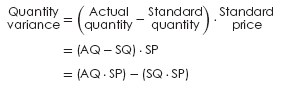Below figure shows a general model [a three-column model] for variance analysis that incorporates items (1), (2), and (3) from the above equations.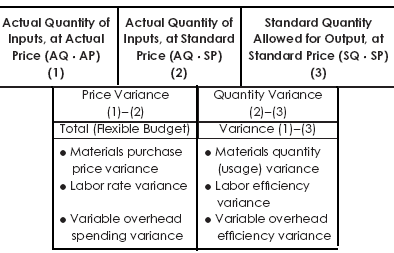It is important to make four observations

1. A price variance and a quantity variance can be calculated for all three variable cost items—direct materials, direct labor, and the variable portion of factory overhead. The variance is not called by the same name, however. For example, a price variance is called a materials price variance in the case of direct materials, but a labor rate variance in the case of direct labor and a variable overhead spending variance in the case of variable factory overhead.
2. A cost variance is unfavorable (U) if the actual price (AP) or actual quantity (AQ) exceeds the standard price (SP) or standard quantity SQ; a variance is favorable (F)” if the actual price or actual quantity is less than the standard price or standard quantity.
3. The standard quantity allowed for output—item (3)—is the key concept in variance analysis. This is the standard quantity that should have been used to produce actual output. It is computed by multiplying the actual output by the number of input units allowed.
4. Variances for fixed overhead are of questionable usefulness for control purposes, since these variances are usually beyond the control of the production department.

Next, we will illustrate the variance analysis for each of the variable manufacturing cost items. Move on…

How Are Material Variances Computed?

A materials purchase price variance is isolated at the time the material is purchased. It is computed based on the actual quantity purchased. The “purchasing department” is responsible for any materials price variance that might occur. The materials quantity (usage) variance is computed based on the actual quantity used. The “production department” is responsible for any materials quantity variance.

Unfavorable price variances may be caused by: inaccurate standard prices, inflationary cost increases, scarcity in raw material supplies resulting in higher prices, and purchasing department inefficiencies. Unfavorable material quantity variances may be explained by poorly trained workers, by improperly adjusted machines, or by outright waste on the production line.

EXAMPLE  Lie Dharma Corp. uses a standard cost system.

The standard variable costs for product are as follows:

Materials: 2 pounds at \$3 per pound
Labor: 1 hour at \$5 per hour
Variable overhead: 1 hour at \$3 per hour

During March, 25,000 pounds of material were purchased for \$74,750, and 20,750 pounds of material were used in producing 10,000 units of finished product. Direct labor costs incurred were \$49,896 (10,080 direct labor hours), and variable overhead costs incurred were \$34,776.

Using the general model [three-column model], the materials variances are shown on the figure below. It is important to note that the amount of materials purchased [25,000 pounds] differs from the amount of materials used in production [20,750 pounds]. The materials purchase price variance was computed using 25,000 pounds purchased, whereas the materials quantity [usage] variance was computed using the 20,750 pounds used in production. A total variance cannot be computed because of the difference.

Alternatively, we can compute the materials variances as:

Materials purchase price variance = AQ (AP – SP)
= (AQ • AP) – (AQ • SP)
= (25,000 pounds) (\$2.99 – \$3.00)
= \$74, 750 –  \$75, 000
= \$250 (Favorable)

Materials quantity (usage) variance
= (AQ – SQ) SP
= (20,750 pounds – 20,000 pounds) (\$3.00)
= \$62, 250 – \$60, 000
= \$2, 250(Unfavorable)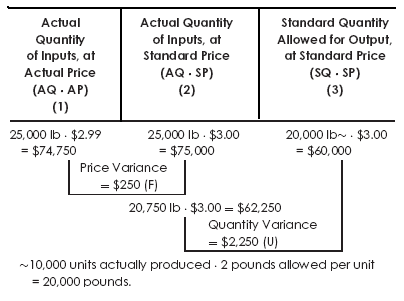How Are Labor Variances Computed?

Labor variances are isolated when labor is used for production. They are computed in a manner similar to the materials variances, except that in the three-column model the terms efficiency and rate are used in place of the terms quantity and price. The production department is responsible for both the prices paid for labor services and the quantity of labor services used. Therefore, the production department must explain why any labor variances occur.

Unfavorable rate variances may be explained by an increase in wages, or the use of labor commanding higher wage rates than contemplated. Unfavorable efficiency variances may be explained by poor supervision, poor quality workers, poor quality of materials requiring more labor time, or machine breakdowns.

Alternatively, we can calculate the labor variances as: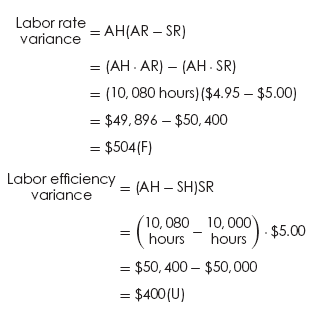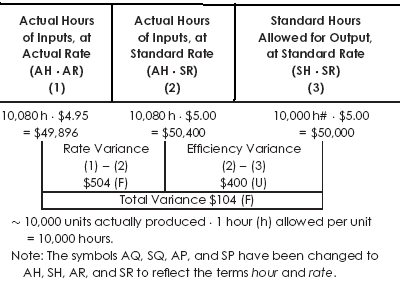How Are Variable Overhead Variances Computed?

The variable overhead variances are computed in a way very similar to the labor variances. The production department is usually responsible for any variable overhead variance.

Unfavorable variable overhead spending variances may be caused by a large number of factors: acquiring supplies for a price different from the standard, using more supplies than expected, waste, and theft of supplies.

Unfavorable variable overhead efficiency variances might be caused by such factors as poorly trained workers, poor-quality materials, faulty equipment, work interruptions, poor production scheduling, poor supervision, employee unrest, and so on.

When variable overhead is applied using direct labor hours, the efficiency variance will be caused by the same factors that cause the labor efficiency variance. However, when variable overhead is applied using machine hours, inefficiency in machinery will cause a variable overhead efficiency variance.

EXAMPLE Using the same data given in Example, we can compute the variable overhead variances.

Alternatively, we can compute the variable overhead variances as: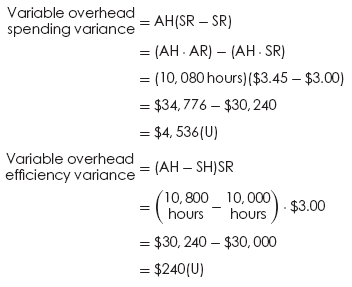Are you looking for easy accounting tutorial? Established since 2007, Accounting-Financial-Tax.com hosts more than 1300 articles (still growing), and has helped millions accounting student, teacher, junior accountants and small business owners, worldwide.